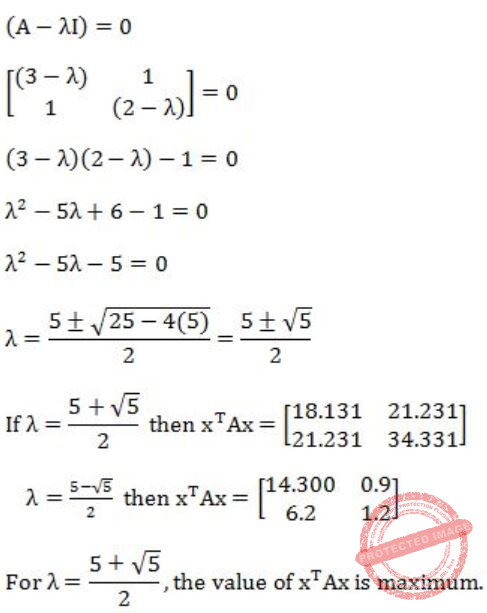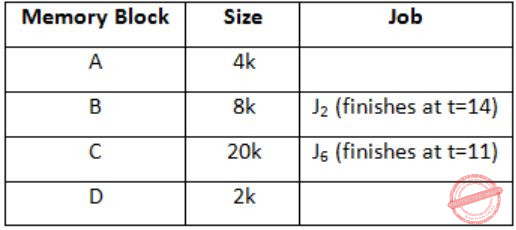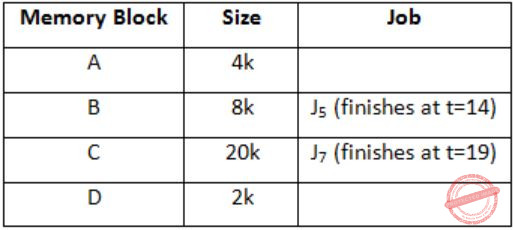## GATE 2007-IT

 Question 1

Suppose there are two coins. The first coin gives heads with probability 5/8 when tossed, while the second coin gives heads with probability 1/4. One of the two coins is picked up at random with equal probability and tossed. What is the probability of obtaining heads ?

 A 7/8 B 1/2 C 7/16 D 5/32
Engineering-Mathematics       Probability
Question 1 Explanation:
Each coin has equal probability to pick i.e., 1/2.
= (1/2)(5/8) + (1/2)(1/4)
= (5/16) + (1/8)
= 7/16
 Question 2

Let A be theWhat is the maximum value of xTAx where the maximum is taken over all x that are the unit eigenvectors of A?

 A 5 B 5+√5/2 C 3 D 5-√5/2
Engineering-Mathematics       Linear-Algebra
Question 2 Explanation:Question 3

Consider a weighted undirected graph with positive edge weights and let uv be an edge in the graph. It is known that the shortest path from the source vertex s to u has weight 53 and the shortest path from s to v has weight 65. Which one of the following statements is always true?

 A weight (u, v) < 12 B weight (u, v) ≤ 12 C weight (u, v) > 12 D weight (u, v) ≥ 12
Algorithms        Shortest Path
Question 3 Explanation:
weight (u,v) ≥ 12
Lets check other options,
A) If the weight (u,v) < 12
Minimum weight of (s,v)
= weight of (s,v) + weight of (u,v)
= 53 + (<12)
= less than 65, which is not possible because shortest path from s to v has weight 65.
Similarly, we can check for option (B) and (C).
 Question 4

In the Spiral model of software development, the primary determinant in selecting activities in each iteration is

 A Iteration size B Cost C Adopted process such as Rational Unified Process or Extreme Programming D Risk
Software-Engineering       Software-process-models
Question 4 Explanation:
Note: Out of syllabus.
 Question 5

Which of the following systems is a most likely candidate example of a pipe and filter architecture ?

 A Expert system B DB repository C Aircraft flight controller D Signal processing
Signal-processing       pipe-and-filter-architecture
Question 5 Explanation:
Note: Out of syllabus.
 Question 6

A processor takes 12 cycles to complete an instruction I. The corresponding pipelined processor uses 6 stages with the execution times of 3, 2, 5, 4, 6 and 2 cycles respectively. What is the asymptotic speedup assuming that a very large number of instructions are to be executed?

 A 1.83 B 2 C 3 D 6
Computer-Organization       Pipelining
Question 6 Explanation:
Let there be n instructions.
For a non-pipelined processor each instruction takes 12 cycles.
So for n instructions total execution time be 12 × n = 12n
For a pipelined processor each instruction takes
max (3, 2, 5, 4, 6, 2) = 6
So for n instructions total execution time be,
(1×6 + (n-1) × 1) × 6
= (6 + n - 1) × 6
= (5 + n) × 6
= 30 + 6n
∴ Speedup = time without pipeline/time with pipeline = 12n/30+6n
So, if n is very large,Question 7

Which of the following input sequences for a cross-coupled R-S flip-flop realized with two NAND gates may lead to an oscillation?

 A 11, 00 B 01, 10 C 10, 01 D 00, 11
Digital-Logic-Design       Sequential-Circuits
Question 7 Explanation:
RS slip-flop using NAND gates.
So, 00 input cause indeterminate state which may lead to oscillation.
 Question 8

The following circuit implements a two-input AND gate using two 2-1 multiplexers. What are the values of X1, X2, X3 ?A X1 = b, X2 = 0, X3 = a B X1 = b, X2 = 1, X3 = b C X1 = a, X2 = b, X3 = 1 D X1 = a, X2 = 0, X3 = b
Digital-Logic-Design       Multiplexer
Question 8 Explanation:
F = (bX1' + aX1)X3 + X2X3'
If we put
X1 = b
X2 = 0
X3 = a
Then we get,
F = ab
 Question 9

Consider an ambiguous grammar G and its disambiguated version D. Let the language recognized by the two grammars be denoted by L(G) and L(D) respectively. Which one of the following is true ?

 A L (D) ⊂ L (G) B L (D) ⊃ L (G) C L (D) = L (G) D L (D) is empty
Compiler-Design       Grammar
Question 9 Explanation:
By changing the corresponding grammar, the language will not be changed.
For example, by converting NFA to DFA language will not be changed.
 Question 10

Processes P1 and P2 use critical_flag in the following routine to achieve mutual exclusion. Assume that critical_flag is initialized to FALSE in the main program.

```        get_exclusive_access ( )
{
if (critical _flag == FALSE) {
critical_flag = TRUE ;
critical_region () ;
critical_flag = FALSE;
}
}```

Consider the following statements. i. It is possible for both P1 and P2 to access critical_region concurrently. ii. This may lead to a deadlock.

Which of the following holds?

 A (i) is false and (ii) is true B Both (i) and (ii) are false C (i) is true and (ii) is false D Both (i) and (ii) are true
Operating-Systems       Process-Synchronization
Question 10 Explanation:
Both the processes run concurrently if they run concurrently till the execution then there is no deadlock.
 Question 11

Let a memory have four free blocks of sizes 4k, 8k, 20k, 2k. These blocks are allocated following the best-fit strategy. The allocation requests are stored in a queue as shown below.The time at which the request for J7 will be completed will be

 A 16 B 19 C 20 D 37
Operating-Systems       Process-Scheduling
Question 11 Explanation:
At t = 0At t = 8At t = 10At t = 11J7 can be finished at t = 19.
 Question 12

The address sequence generated by tracing a particular program executing in a pure demand paging system with 100 bytes per page is

` 0100, 0200, 0430, 0499, 0510, 0530, 0560, 0120, 0220, 0240, 0260, 0320, 0410. `
Suppose that the memory can store only one page and if x is the address which causes a page fault then the bytes from addresses x to x + 99 are loaded on to the memory. How many page faults will occur?

 A 0 B 4 C 7 D 8
Operating-Systems       Demand-Paging
Question 12 Explanation:
0100 - Page fault, address till 199 in memory
0200 - Page fault, address till 299 in memory
0430 - Page fault, address till 529 in memory
0499 - No page fault
0510 - No page fault
0530 - Page fault, address till 629 in memory
0560 - No page fault.
0120 - Page fault, address till 219 in memory
0220 - Page fault, address till 319 in memory
0240 - No page fault
0260 - No page fault
0320 - Page fault, address till 419 in memory
0410 - No page fault
So, total page faults = 7.
 Question 13

Consider the following statements about the timeout value used in TCP.
(i) The timeout value is set to the RTT (Round Trip Time) measured during TCP connection establishment for the entire duration of the connection.
(ii) Appropriate RTT estimation algorithm is used to set the timeout value of a TCP connection.
(iii) Timeout value is set to twice the propagation delay from the sender to the receiver.
Which of the following choices hold?

 A (i) is false, but (ii) and (iii) are true B (i) and (iii) are false, but (ii) is title C (i) and (ii) are false, but (iii) is true D (i), (ii) and (iii) are false
Computer-Networks       TCP
Question 13 Explanation:
Statement-I: It is False.
The timeout value cannot be fixed for entire duration as it will turn timer to static timer, we need dynamic timer for timeout.
Statement-II: It is True.
Basic algorithm, Jacobson's algorithm, Karl's modification; these three algorithms are to be appropriate to RTT estimation algorithm used to set timeout value dynamically.
Statement-III: It is False.
Because timeout value is set to twice the propagation delay in data link layer where hop to hop distance is known, not in TCP layer.
 Question 14

Consider a TCP connection in a state where there are no outstanding ACKs. The sender sends two segments back to back. The sequence numbers of the first and second segments are 230 and 290 respectively. The first segment was lost, but the second segment was received correctly by the receiver. Let X be the amount of data carried in the first segment (in bytes), and Y be the ACK number sent by the receiver. The values of X and Y (in that order) are

 A 60 and 290 B 230 and 291 C 60 and 231 D 60 and 230
Computer-Networks       TCP
Question 14 Explanation:
In the 1st segment data is from byte number 230 to byte number 289, that is 60 bytes. As 1st segment is lost, so TCP will send ACK for the next-in-order segment receiver is to be expecting. So, it will be for 230.
 Question 15

Consider the following two statements:
(i) A hash function (these are often used for computing digital signatures) is an injective function.
(ii) An encryption technique such as DES performs a permutation on the elements of its input alphabet.
Which one of the following options is valid for the above two statements?

 A Both are false B Statement (i) is true and the other is false C Statement (ii) is true and the other is false D Both are true
Computer-Networks       Network-Security
Question 15 Explanation:
i) Hash function is many to one function. It is not one-one (or) injective.
ii) It uses the P-Box permutation.
Statement-I is false, II is true.
 Question 16

The minimum positive integer p such that 3p modulo 17 = 1 is

 A 5 B 8 C 12 D 16
Engineering-Mathematics       Numerical-Methods
Question 16 Explanation:
According to Fermat's little theorem,
ap-1 mod p = 1
Here, p = 17
So, p-1 = 16 is the answer.
 Question 17

Exponentiation is a heavily used operation in public key cryptography. Which of the following options is the tightest upper bound on the number of multiplications required to compute bn mod m, 0≤b, n≤m?

 A O(log n) B O(√n) C O(n/log n) D O(n)
0       Algorithms
Question 17 Explanation:
In this we need to compute like
C1 = bn/2 × bn/2
C2 = bn/4 × bn/4
C3 = bn/8 × bn/8

Ck = b2 × b2
Recurrence relation T(n) = T(n/2) + O(1)
T(n) = O(log n)
 Question 18

A firewall is to be configured to allow hosts in a private network to freely open TCP connections and send packets on open connections. However, it will only allow external hosts to send packets on existing open TCP connections or connections that are being opened (by internal hosts) but not allow them to open TCP connections to hosts in the private network. To achieve this the minimum capability of the firewall should be that of

 A A combinational circuit B A finite automaton C A pushdown automaton with one stack D A pushdown automaton with two stacks
Computer-Networks       TCP
Question 18 Explanation:
Pushdown automata with two stacks which is the Turing machine.
Turing machine can do everything as the normal computer can do, so firewall can be created by the TM.
 Question 19

Given below are HTML lines,With reference to the HTML lines given above, consider the following statements.
(i) Clicking on the point <80, 75> does not have any effect.
(ii) The web browser can identify the area applicable to the mouse-click within the image and the subsequent action to be taken without additional responses from the web server.
(iii) The dots in the cgi-bin URL will be resolved by the web browser before it is sent to the web server.
(iv) The "fd.html" request when sent to the web server will result in a GET request.
Exactly how many of the statements given above are correct?

 A 0 B 1 C 2 D 3
Web-Technologies       HTML
Question 19 Explanation:
Note: Out of syllabus.
 Question 20

Consider the XML document fragment given below:Consider the XPath expression: *[not (self)::TOC]
What would be the result of the given XPath expression when the current node is Book?

 A The Title and Content elements B The Content and TOC elements C The Title and TOC elements D The Title, Content and TOC elements
Web-Technologies       XML
Question 20 Explanation:
Note: Out of syllabus.
 Question 21

Which one of these first-order logic formula is valid?

 A ∀x(P(x) ⇒ Q(x)) ⇒ (∀xP(x) ⇒ ∀xQ(x)) B ∃x(P(x) ∨ Q(x)) ⇒ (∃xP(x) ⇒ ∃xQ(x)) C ∃x(P(x) ∧ Q(x)) (∃xP(x) ∧ ∃xQ(x)) D ∀x∃y P(x, y) ⇒ ∃y∀x P(x, y)
Engineering-Mathematics       Propositional-Logic
Question 21 Explanation:
LHS = for every x, if P holds then Q holds
RHS = if P(x) holds for all x, then Q(x) holds for all x
LHS ⇒ RHS (✔)
RHS ⇒ LHS (️×)
There are 21 questions to complete.

Register Now